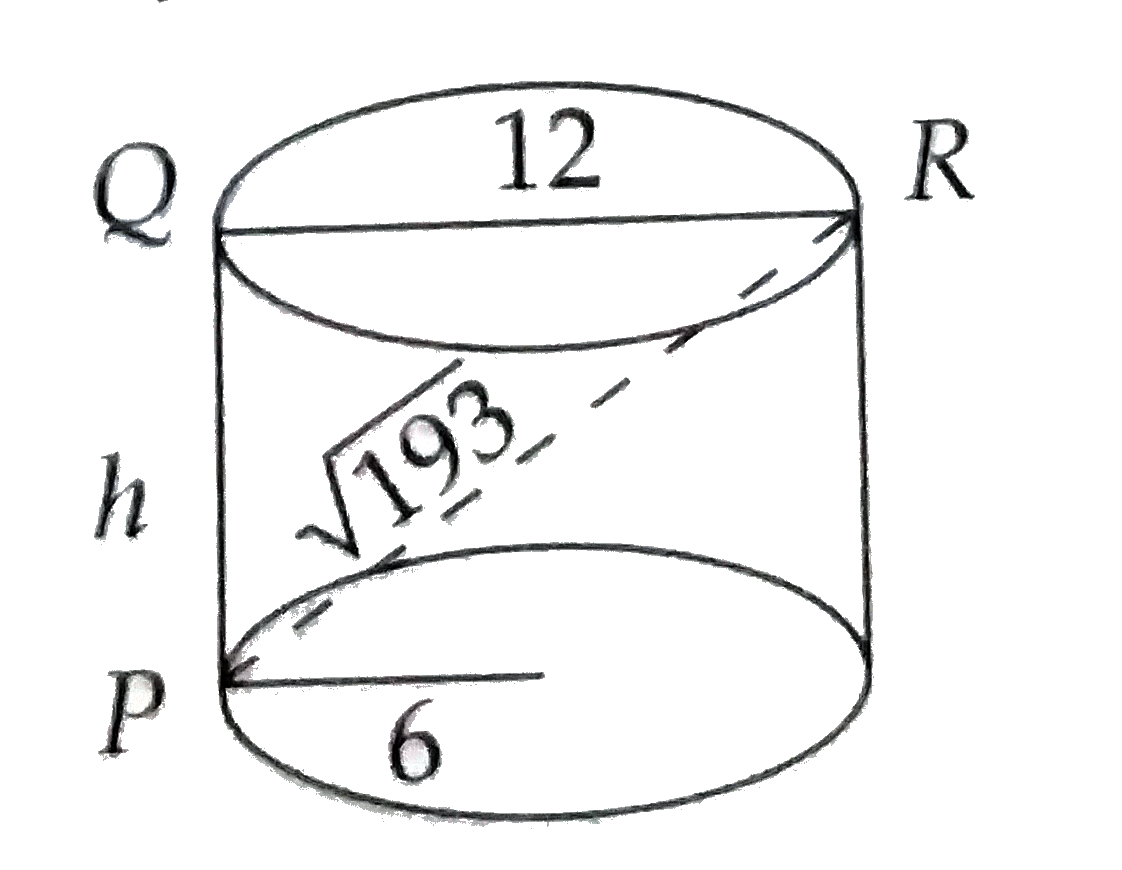# The greatest possible distance between any two points on the surface of a right circular cylinder is √193 and the area of the circular base of the right circular cylinder is 36π. What is the volume of the right circular cylinder ?Khareedo DN Pro and dekho sari videos bina kisi ad ki rukaavat ke!

##### Updated On: 27-06-2022

Text Solution

A
252π
B
294π
C
343π
D
386π
Solution
The volume of a right circular cylinder having a radius r and a height h is πr2h. To find the volume of this right circular cylinder, you need its radius and its height. Now the area of a circle with a radius r is πr2. The area of the circular base is 36π. If the radius of the circular base is r, then πr2=36π,r2=36, and r = 6. You now have the radius. Thediameter is 2(6) = 12. Let's call the height of the right circular cylinder h and draw a picture.The greatest possible distance between two points on the surface of the right circular cylinder is the distance between points P and R. Use the Pythagorean theorem in right triangle PQR to find the height h.
122+h2=(193)2
144+h2=193
h2=49
h = 7
The volume of the right circular cylinder is πr2h=π(62)(7)=π(36)(7)=252π.